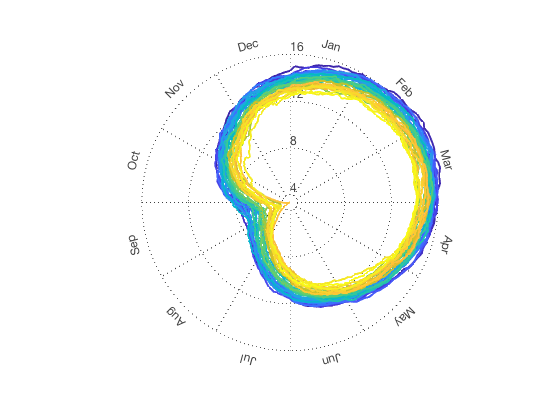# spiralplot documentation

The spiralplot function makes a spiral plot that shows the annual nature and long-term trend of a time series.

This function was inspired by work by Ed Hawkins of the University of Reading. More information on spiral plots can be found here.

Back to Climate Data Tools Contents

## Syntax

```spiralplot(t,z)
spiralplot(...,'LineWidth',LineWidth)
spiralplot(...,'zmin',zmin)
spiralplot(...,'ztick',Zticks)
spiralplot(...,'format',MonthFormat)
spiralplot(...,'fontsize',LabelFontsize)
spiralplot(...,'nospokes')
[h,hax,htxt] = spiralplot(...)```

## Description

spiralplot(t,z) plots a time series of z as a spiral plot, where time is in datetime or datenum format.

spiralplot(...,'LineWidth',LineWidth) specifies LineWidth of the spiral plot. Default LineWidth is 2.

spiralplot(...,'zlim',zlim) specifies a minimum and maximum values the z axis. Default zlim corresponds to the range of z; however, depending on the application it might make sense to put 0 or some other critical value at the center of the graph.

spiralplot(...,'ztick',Zticks) specifies the values of z axis lines and labels.

spiralplot(...,'format',MonthFormat) formats the way months are labeled using datestr formatting options. Options for month formatting are:

• 'mmmm' Full name, (e.g., March, December)
• 'mmm' First three letters, (e.g., Mar, Dec)
• 'mm' Two digits, (e.g., 03, 12)
• 'm' Capitalized first letter, (e.g., M, D)

spiralplot(...,'fontsize',LabelFontsize) specifies the fontsize of the month names and z axis labels.

spiralplot(...,'nospokes') removes the spokes from the axes.

[h,hax,htxt] = spiralplot(...) returns handles of the color-scaled line, axis objects, and text objects.

## Example 1: Simple

This example uses sea ice extent data from the NSIDC (See References below).

Load the example data and plot the northern hemisphere sea ice extent:

```load seaice_extent

spiralplot(t,extent_N)
```## Example 2: Specify z axis limits

The plot above does not show how close to zero the sea ice extent has been. So it might be best to put zero in the center of the circle:

```figure
spiralplot(t,extent_N,'zlim',[0 18])
```## Example 3: Specify z ticks:

Perhaps you want to label the values 3, 8.2, and 15 instead of using the default tick label calculations. Here's how:

```figure
spiralplot(t,extent_N,'zlim',[0 18],'ztick',[3 8.2 15])
```## Example 4: Add a colorbar for time

To include a colorbar that shows time, use the cbdate function.

```cb = colorbar('location','southoutside');
cbdate('yyyy','horiz')
```## Example 5: Format the axis label text

```cla % clears the spiral plot above, but keeps the colorbar
spiralplot(t,extent_N,'fontsize',8,'format','mmmm')
```## Example 6: Make a gif

Making a gif is pretty easy with spiralplot and the gif function. Just initialize the first frame like this:

`  years = 1980:2017;`
`  figure('position',[100 100 377 420])`
```  % Place a colorbar:
cb = colorbar('southoutside');
set(cb,'fontsize',10)```
```  % Set the color axis to the full date range:
caxis(datenum([min(t) max(t)]))```
```  % Format the colorbar axis like dates:
cbdate('yyyy','horiz')```
```  % Get indices of all dates before Jan 1, 1980:
ind = t<datetime(years(1),1,1);```
```  % Make a spiral plot
spiralplot(t(ind),extent_N(ind),'zlim',[0 17],...
'fontsize',10,'format','mmmm')```
```  % Place a text label in the center:
text(0,0,'1979','vert','middle','horiz','center')```
```  % Write the first frame of a new .gif animation:
gif('seaice_extent.gif','frame',gcf)```

After the first frame is set, loop through the rest of the years and save each frame:

```  % Loop through each subsequent year:
for k = 2:length(years)```
`     cla % clears the old plot`
```     % Get indices of all dates before the kth year:
ind = t<datenum(years(k),1,1);```
```     % Make a spiral plot
spiralplot(t(ind),extent_N(ind),'zlim',[0 17],...
'fontsize',10,'format','mmmm')```
```     % Place a text label in the center:
text(0,0,num2str(years(k)-1),...
'vert','middle','horiz','center')```
```     % save this frame:
gif
end```

And here's the result:## References

Sea ice data by Fetterer et al. are available at the NSIDC.

Fetterer, F., K. Knowles, W. Meier, and M. Savoie. 2016, updated daily. Sea Ice Index, Version 2. Boulder, Colorado USA. NSIDC: National Snow and Ice Data Center. doi:10.7265/N5736NV7.

## Author Info

This function was written by Chad A. Greene of the University of Texas Institute for Geophysics (UTIG), June 2017.